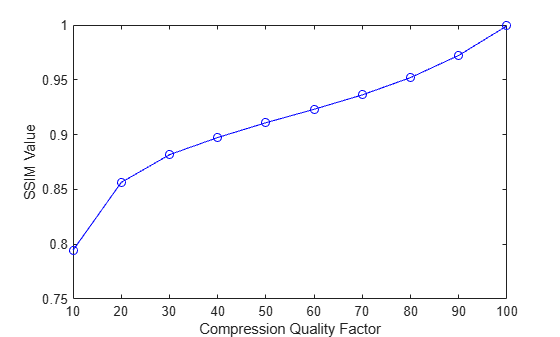# Compare Image Quality at Various Compression Levels

This example shows how to test image quality using `ssim`. The example creates images at various compression levels and then plots the quality metrics. To run this example, you must have write permission in your current folder.

Read an image into the workspace.

`I = imread('cameraman.tif');`

Write the image to a file using various quality values. The JPEG format supports the `'quality'` parameter. Use the `ssim` function to check the quality of each written image.

```ssimValues = zeros(1,10); qualityFactor = 10:10:100; for i = 1:10 imwrite(I,'compressedImage.jpg','jpg','quality',qualityFactor(i)); ssimValues(i) = ssim(imread('compressedImage.jpg'),I); end```

Plot the results. Note how the image quality score improves as you increase the quality value specified with `imwrite`.

```plot(qualityFactor,ssimValues,'b-o'); xlabel('Compression Quality Factor'); ylabel('SSIM Value');```